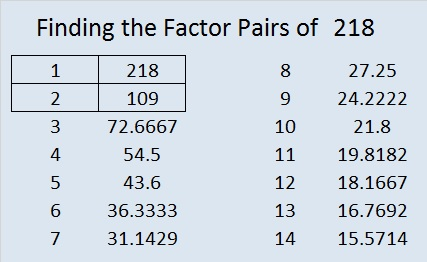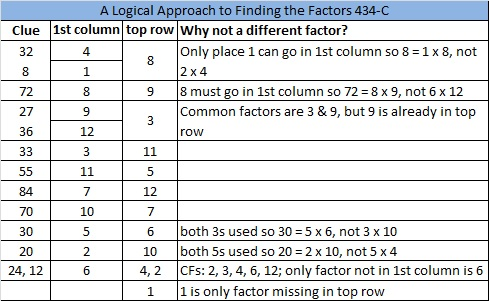# 218 and Level 6

• 218 is a composite number.
• Prime factorization: 218 = 2 x 109
• The exponents in the prime factorization are 1 and 1. Adding one to each and multiplying we get (1 + 1) x (1 + 1) = 2 x 2 = 4. Therefore 218 has 4 factors.
• Factors of 218: 1, 2, 109, 218
• Factor pairs: 218 = 1 x 218 or 2 x 109
• 218 has no square factors so its square root cannot be simplified. √218 ≈ 14.765Excel file of puzzles and previous week’s factor solutions: 12 Factors 2014-08-25This site uses Akismet to reduce spam. Learn how your comment data is processed.